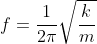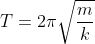# How To Calculate Oscillation: 5 Complete Quick Facts

Everywhere we look, oscillations are occurring. Even the atoms we are made up of are also currently oscillating. So, in this post, we are going to get insight into how to calculate oscillations.

We need to know the time period of an oscillation to calculate oscillations. And from the time period, we will obtain the frequency of oscillation by taking reciprocation of it. The frequency of oscillation will give us the number of oscillations in unit time.

Before taking a deep dig into how to calculate oscillation, we first get a brief idea of oscillation, its cycle, time period, and frequency.

## Physics of Oscillation:

Oscillation is a motion that repeats itself in terms of magnitude or position around its equilibrium or central point along the same path. For example, swinging pendulum, vibrating spring in guitar, etc., are examples of oscillation.

One cycle of oscillation is one complete oscillation, which involves returning to the beginning point and repeating the motion.  To complete this one cycle, whatever time it takes is nothing but its time period. Further, taking its reciprocal, we will have the frequency of oscillation which is considered the number of oscillations over the time period.

Let’s see what is the formula for oscillation now.

## What is the formula for oscillation?

The oscillation formula is just the frequency of the oscillation formula. Thus, we can write it as

When you think of oscillations, the first thing that jumps to mind is a simple harmonic oscillator. So, let’s first get a glimpse of the equation of motion for the simple harmonic oscillator before knowing how to calculate oscillation.

## Equation of motion for SHM (Simple Harmonic Oscillator):

Assume a particle is suspended and oscillating along the Y-axis. As a result, at any given time t, the equation for the position is:

y(t) = A sin⍵t …..(1)

Here, A is the amplitude or the maximum displacement from equilibrium position.

& is the angular frequency of oscillating particle and is given by,

Or

Thus, equation (1) can be written as

y(t) = A sin(2𝜋f)t …..(2)

The first derivative of equation (2) will give the particle’s velocity, while the second derivative will give the particle’s acceleration.

Thus,

v(t) = 2𝜋fA cos(2𝜋ft) …..(3)

a(t) = -(2𝜋f)2 [A sin(2𝜋ft)] …..(4)

Putting value of equation (2) in (4), we will get,

a(t) = -(2𝜋f)2 y(t) …..(5)

∴ a = -4𝜋2f2 y …..(6)

So, if we want to figure out how to calculate oscillation, we’ll look at two different scenarios: a spring-mass system and a pendulum.

## ➠ How to calculate oscillation of a spring mass system:

Consider a mass suspended to one end of spring, as shown in the figure below. Due to the weight of the mass, the spring will be stretched by distance y. Thus, the upward pull of spring as per Hooke’s law is:

F = -ky

where k is spring constant, which measures spring’s stiffness, and y is stretch in the spring.

In the spring mass system, the spring force is upward, and the gravitational force is downward. When both forces balance each other, the mass becomes motionless. Thus, we can write:

mg =-ky

As g is gravitational acceleration, putting the value of equation (6) in the above equation, we get,

∴m(-4𝜋2f2y) = -ky

Making frequency f subject of the equation we get…..(7)

And thus time period can be given by:…..(8)

After spring mass system now let’s see how to calculate oscillation of pendulum.

## ➠ How to calculate oscillation of pendulum:

To calculate the oscillation of the pendulum, we will consider three cases:

• Simple pendulum
• Physical or Compound pendulum
• Torsional pendulum

## ✽ Oscillations of the simple pendulum:

A simple pendulum is made up of a string attached to the mass m. As shown in the figure below, if the bob of mass m moves away from equilibrium at an angle 𝜃, then the restoring force brings it back to the equilibrium position. The bob is subjected to the forces of gravity and string tension. Tension on the spring is balanced by the cos component of bob weight. The restoring force here in the pendulum is due to the tangential component or the sin component of gravitational force.

Thus, magnitude of restoring force is,

|F| = mgsin𝜃

Bob will be able to accelerate due to the restoring force. As a result, according to Newton’s second law,

ma = F = -mgsin𝜃

where the negative sign shows here that restoring force is in the opposite direction of bob’s motion.

Acceleration will be angular as the motion of the bob is along the arc of the circle. The angular acceleration is given by,

Thus, from the above equations,

But as displacement is small, we can consider sin𝜃 = 𝜃. Thus,

The above equation shows the dependence of angular acceleration on angular displacement. Thus, from equation (6),

𝛼= 4𝜋2f2𝜃

Thus, the frequency and time period of a simple pendulum is:

## ✽ Oscillations of the compound or physical pendulum:

The system formed by suspending a rigid body from a fixed horizontal axis is known as a physical pendulum. If ‘I’ is the moment of inertia of an oscillating rigid body, the frequency and time period of the physical pendulum is:

## ✽ Oscillations of the torsional pendulum:

The system is called a torsional pendulum when an end of the thin wire or rod is attached to the disc-like mass. If ‘I’ is the inertia of the disc, the torsional pendulum’s frequency and period can be expressed as,

Thus, considering all three cases, we can conclude that the frequency and time period of the pendulum are dependent on the length of the cord and independent of the body’s mass. You might be wondering how to calculate the time period of oscillation practically. So let’s see how is oscillation time calculated.

## How is oscillation time calculated?

The time period of an oscillation can be calculated both experimentally and mathematically.

The method of the experiment of the spring mass system and pendulum is almost the same. The difference is that you need not find out the spring constant as we are not using any spring in the pendulum.

• To calculate the oscillation of the mass spring system, you need to find the spring constant k. To find spring constant, allow the mass to hang on a spring in a motionless state. Then add additional mass and record change in the spring stretch.

mg -ky =0

• After finding the spring constant, you have to record the time for ten oscillations for each mass. To get an accurate answer to repeat this process thrice. The mean value of these three time periods is considered as the time period of that particular mass. After that, take reciprocal of it, and you get the number of oscillations over the unit time. You can compare your answers by putting values in the respective equations of frequency and time period.

We hope that we have addressed all of your concerns on how to calculate oscillations.

Alpa P. Rajai

I am Alpa Rajai, Completed my Masters in science with specialization in Physics. I am very enthusiastic about Writing about my understanding towards Advanced science. I assure that my words and methods will help readers to understand their doubts and clear what they are looking for. Apart from Physics, I am a trained Kathak Dancer and also I write my feeling in the form of poetry sometimes. I keep on updating myself in Physics and whatever I understand I simplify the same and keep it straight to the point so that it deliver clearly to the readers. You can also reach me at : https://www.linkedin.com/in/alpa-rajai-858077202/